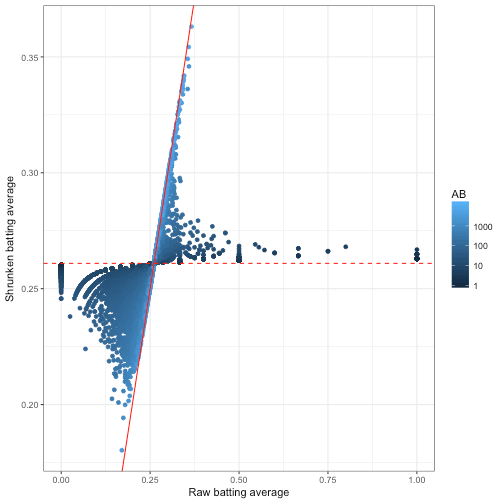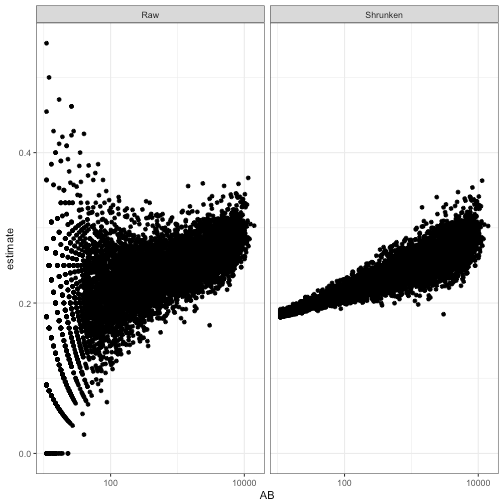Want to share your content on R-bloggers? click here if you have a blog, or here if you don't.

Previously in this series:

We’ve introduced a number of statistical techniques in this series: estimating a beta prior, beta-binomial regression, hypothesis testing, mixture models, and many other components of the empirical Bayes approach. These approaches are useful whenever you have many observations of success/total data.

Since I’ve provided the R code within each post, you can certainly apply these methods to your own data (as some already have!). However, you’d probably find yourself copying and pasting a rather large amount of code, which can take you out of the flow of your own data analysis and introduces opportunities for mistakes.

Here, I’ll introduce the new ebbr package for performing empirical Bayes on binomial data. The package offers convenient tools for performing almost all the analyses we’ve done during this series, along with documentation and examples. This post also serves as review of our entire empirical Bayes series so far: we’ll touch on each post briefly and recreate some of the key results.

### Setup

We start, as always, by assembling some per-player batting data (note that all the code in this post can be found here). It’s worth remembering that while we’re using batting averages as an example, this package can be applied to many types of data.

## Empirical Bayes estimation

In one of the first posts in the series, we noticed that the distribution of player batting averages looked roughly like a beta distribution:We thus wanted to estimate the beta prior for the overall dataset, which is the first step of empirical Bayes analysis. The `ebb_fit_prior` function encapsulates this, taking the data along with the success/total columns and fitting the beta through maximum likelihood.

`prior` is an `ebb_prior` object, which is a statistical model object containing the details of the beta distribution. Here we can see the overall alpha and beta parameters. (We’ll see later that it can store more complicated models).

The second step of empirical Bayes analysis is updating each observation based on the overall statistical model. Based on the philosophy of the broom package, this is achieved with the augment function:

Notice we’ve now added several columns to the original data, each beginning with `.` (which is a convention of the `augment` verb to avoid rewriting e. We have the `.alpha1` and `.beta1` columns as the parameters for each player’s posterior distribution, as well as `.fitted` representing the new posterior mean (the “shrunken average”).

We often want to run these two steps in sequence: estimating a model, then using it as a prior for each observation. The `ebbr` package provides a shortcut, combining them into one step with `add_ebb_estimate`:

(The `add_` prefix is inspired by modelr’s `add_residuals` and `add_predictions`, which also fit a statistical model then append columns). Note the `prior_subset` argument, which noted that while we wanted to keep all the shrunken values in our output, we wanted to fit the prior only on individuals with at least 500 at-bats.

### Estimates and credible intervals

Having the posterior estimates for each player lets us explore the model results using our normal tidy tools like dplyr and ggplot2. For example, we could visualize how batting averages were shrunken towards the mean of the prior:This was one of our first visualizations in the empirical Bayes estimation post. I like how it captures what empirical Bayes estimation is doing: moving all batting averages towards the prior mean (the dashed red line), but moving them less if there is a lot of information about that player (high AB).

In the following post, we used credible intervals, to visualize our uncertainty about each player’s true batting average. The output of `add_ebb_estimate` comes with those credible intervals in the form of `.low` and `.high`. This makes it easy to visualize these intervals for particular players, such as the 1998 Yankees:Notice that once we have the output from `add_ebb_estimate`, we’re no longer relying on the `ebbr` package, only on dplyr and ggplot2.

## Hierarchical modeling

In two subsequent posts, we examined how this beta-binomial model may not be appropriate, because of the relationship between a player’s at-bats and their batting average. Good batters tend to have long careers, while poor batters may retire quickly.We solved this by fitting a prior that depended on AB, through beta-binomial regression. The `add_ebb_estimate` function offers this option, by setting `method = "gamlss"` and providing a formula to `mu_predictors`.

(You can also provide `sigma_predictors` to have the variance of the beta depend on regressors, though it’s less common that you’d want to do so).

The augmented output is now a bit more complicated: besides the posterior parameters such as `.alpha1`, `.beta1`, and `.fitted`, each observation also has its own prior parameters `.alpha0` and `.beta0`. These are predicted based on the regression on `AB`.

The other parameters, such as `.fitted` and the credible interval, are now shrinking towards a trend rather than towards a constant. We can see this by plotting `AB` against the original and the shrunken estimates:As we saw in the post on hierarchical modeling, the model can incorporate still more useful information. For example, it could include what year they play in, and whether they are left- or right- handed, both of which tend to affect batting average.

In this case I’m fitting the prior with `ebb_fit_prior` rather than adding the estimates with `add_ebb_estimate`. This lets us feed it new data that we generate ourselves, and examine how the posterior distribution would change. This takes a bit more work, but lets us re-generate one of the more interesting plots from that post about how time and handedness relate:Since the `ebbr` package makes these models convenient to fit, you can try a few variations on the model and compare them.

## Hypothesis testing

Another pair of posts examined the problem of hypothesis testing. For example, we wanted to get a posterior probability for the statement “this player’s true batting average is greater than .300”, so that we could construct a “Hall of Fame” of such players.

This method is implemented in the `add_ebb_prop_test` (notice that like `add_ebb_estimate`, it adds columns to existing data). `add_ebb_prop_test` takes the output of an earlier `add_ebb_estimate` operation, which contains posterior parameters for each observation, and appends columns to it:

(Note the `sort = TRUE` argument, which sorts in order of our confidence in each player). There are now too many columns to read easily, so we’ll select only a few of the most interesting ones:

Notice that two columns have been added, with per-player metrics described in this post.

• `.pep`: the posterior error probability- the probability that this player’s true batting average is less than .3.
• `.qvalue`: the q-value, which corrects for multiple testing by controlling for false discovery rate (FDR). Allowing players with a q-value below .05 would mean only 5% of the ones included would be false discoveries.

For example, we could find how many players would be added to our “Hall of Fame” with an FDR of 5%, or 1%:

### Player-player A/B test

Another post discussed the case where instead of comparing each observation to a single threshold (like .300) we want to compare to another player’s posterior distribution. I noted that this is similar to the problem of “A/B testing”, where we might be comparing two clickthrough rates, each represented by successes / total.

The post compared each player in history to Mike Piazza, and found players we were confident were better batters. We’d first find Piazza’s posterior parameters:

This vector of two parameters, an alpha and a beta, can be passed into `add_ebb_prop_test` just like we passed in a threshold:1

Again we select only a few interesting columns:

Just like the one-sample test, we’ve added `.pep` and `.qvalue` columns. From this we can see a few players who we’re extremely confident are better than Piazza.

### Mixture models

This brings us to my recent post on mixture models, where we noticed that when pitchers are included, the data looks a lot less like a beta distribution and more like a combination of two betas.Fitting a mixture model, to separate out the two beta distributions so they could be shrunken separately, took a solid amount of code.

The `ebbr` package thus provides tools for fitting a mixture model using an iterative expectation-maximization algorithm, with the `ebb_fit_mixture` function. Like the other estimation functions, it takes a table as the first argument, followed by two arguments for the “successes” column and the “total” column:

It returns the parameters of two (or more) beta distributions:

It also assigns each observation to the most likely cluster. Here, we can see that cluster 1 is made up of pitchers, while cluster 2 is the non-pitchers:### Mixture model across iterations

You may be interested in how the mixture model converged to its parameters. The `iterations` component of the `ebb_mixture` object contains details on the iterations, which can be visualized.Note that it took only about one full iteration for the parameters to get pretty close to their eventual values. We can also examine the change in cluster assignments for each observation:The package’s functions for mixture modeling are a bit more primitive than the rest: I’m still working out the right output format, and some of the details are likely to change (this is one of the reasons I haven’t yet submitted `ebbr` to CRAN). Still, the package makes it easier to experiment with expectation-maximization algorithms.

## What’s Next: Simulation

We’re approaching the end of this series on empirical Bayesian methods, and have touched on many statistical approaches for analyzing binomial data, all with the goal of estimating the “true” batting average of each player. There’s one question we haven’t answered, though: do these methods actually work?

Even if we assume each player has a “true” batting average as our model suggests, we don’t know it, so we can’t see if our methods estimated it accurately. We believe that empirical Bayes shrinkage gets closer to the true probabilities than raw batting averages do, but we can’t measure its bias or mean-squared error. We can’t see whether credible intervals actually contain the true parameters, or whether our FDR control was successful in finding a set of players above a cutoff. This means we can’t test our methods, or examine when they work well and when they don’t.

In the next post, we’ll simulate some fake batting average data, which will let us know the true probabilities for each player, then examine how close our statistical methods got. Simulation is a universally useful way to test a statistical method, to build intuition about its mathematical properies, and to gain confidence that we can trust its results. This will be especially easy now that we have the `ebbr` package to encapsulate the methods themselves, and will be a good chance to demonstrate the tidyverse approach to simulation.

1. This is rather similar to how the built-in prop.test function handles the difference between one-sample and two-sample tests. I’ve often found this kind of behavior annoying (determining what test to use based on the length of the input), but I must admit it is convenient.